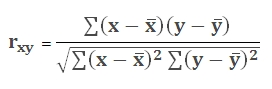# SQL Server PEARSON function

Updated: 9 August 2010

Use PEARSON_q to calculate the product moment correlation coefficient between two datasets. The equation for the Pearson correlation coefficient isSyntax
SELECT [wctStatistics].[wct].[PEARSON_q] (
<@Known_y_Known_x_RangeQuery, nvarchar(4000),>)
Arguments
@Known_y_Known_x_RangeQuery
the select statement, as text, used to determine the known y- and x-values to be used in the PEARSON_q calculation.
Return Types
float
Remarks
·         If the number of known-x data points is different than the number of known-y data points, PEARSON_q will return an error
·         If the standard deviation of either set of data points is zero, then PEARSON_q will return an error.
·         This function works with normalized and de-normalized tables. For simpler queries consider using PEARSON.
·         No GROUP BY is required for this function even though it produces aggregated results.
Examples

To determine the Pearson coefficient between grades in math and grades in science:
from #s1 a, #s1 b
where a.subject = ' + Char(39) + 'Math' + Char(39) + ' and
b.subject = ' + Char(39) + 'Science' + Char(39) + 'and
a.student = b.student')

This produces the following result

----------------------
0.999999494275124

(1 row(s) affected)

To determine the correlation between grades in math and grades in science on a de-normalized table:

SELECT wct.PEARSON_q('SELECT math, science from #s2')

This produces the following result

----------------------
0.999999494275124

(1 row(s) affected)

### SupportCopyright 2008-2023 Westclintech LLC         Privacy Policy        Terms of Service